Higher-Order Simulations: Strategic Investment Under Model-Induced Price Patterns

Journal of Artificial Societies and Social Simulation vol. 10, no. 2, 6
<http://jasss.soc.surrey.ac.uk/10/2/6.html>

Received: 24-Feb-2006    Accepted: 03-Mar-2007    Published: 31-Mar-2007###Abstract

The trading and investment decision processes in financial markets become ever more dependent on the use of valuation and risk models. In the case of risk management for instance, modelling practice has become quite homogeneous and the question arises as to the effect this has on the price formation process. Furthermore, sophisticated investors who have private information about the use and characteristics of these models might be able to make superior gains in such an environment. The aim of this article is to test this hypothesis in a stylised market, where a strategic investor trades on information about the valuation and risk management models used by other market participants. Simulation results show that under certain market conditions, such a 'higher-order' strategy generates higher profits than standard fundamental and momentum strategies that do not draw on information about model use.

Keywords:
Financial Markets, Multi-Agent Simulation, Performativity, Higher-Order Strategies

###Introduction

#### Context and Scope

1.1
Recent advances in financial mathematics and the ready availability of almost unlimited computer power have led to an explosive growth in the development and use of simulation techniques in financial practice. Investors, traders, and fund managers have grown accustomed to back up investment decisions with expert advice emanating from a wide range of valuation models and risk management systems. More so, hedge funds and proprietary desks incorporate sophisticated statistical and stochastic models into automatic trading systems to scavenge financial markets for lucrative investments opportunities.

1.2
As the use of mathematical models for valuation, forecasting, and risk measurement becomes more pervasive, the question arises as to its effect on market practice and price dynamics. Has the financial modelling practice the potential to substantially interfere with the price formation process in any systematic way? Or should one rather accept the traditional view that financial models are based on mere descriptions of observed market phenomena, and that their use has no predictable effect on price formation? The causal efficacy, or performativity, of financial models has been discussed extensively in the Social Studies of Finance literature (MacKenzie 2006) and revolves around the question whether and under what circumstances financial models are instrumental in shaping the market reality they are describing. We focus here on a particular implication of the performativity thesis in the context of strategic trading. For investment decisions that depend to a great extent on valuation and risk management strategies, performativity entails the possibility of regularities in price patterns that can in principle be exploited by informed traders. In the right circumstances, these regularities will become more pronounced the more investors adopt financial models that are based on identical assumptions and use the same mathematics.

1.3
In the remaining part of this article, we will test this hypothesis in a stylised market setting similar to that of Farmer (1998), safe for the addition of a strategic trader who has privileged information of the valuation and risk management models used by fundamental and noise traders. Consequently, as the increased use of models causes the decision making problem to become more 'regular', strategic traders can exploit the situation by selecting strategies that account for the pervasive use of financial models.

#### Exploiting Counterperformativity

1.4
In a recent article on the sociology of arbitrage trading, MacKenzie (2003) suggests that during the 1998 liquidity crisis, modern risk management practice, and notably value-at-risk techniques, induced market interdependencies that lead to heightened price instability — a phenomenon that has also been commented on by policy makers (IMF 1998;BIS 1999;Mayer 1999) — and, in some well-publicised cases, resulted in large trading losses for institutions involved in arbitrage trading. Some commentators have pointed to inaccurate financial models as the principal culprit of recent financial disasters, but it is difficult to see exactly in what way model inaccuracy is linked to price instability in liquid global markets. We believe that a more fruitful avenue was opened with the concept of performativity, a central theme in MacKenzie's sociology of finance. Performativity (counterperformativity) is given when the "practical use of an aspect of economics makes economic processes more (less) like their depiction by economics". As far as risk management is concerned, counterperformativity seems to provide a plausible explanation of how a widespread use of value-at-risk (VaR) models could result in large price movements (Llacay and Peffer 2004). In the present article, we are going to consider the link between the performativity of valuation and risk management models and model-based investment strategies, and analyse how higher-order strategies — namely those that account for these investment strategies — can result in a competitive advantage for informed traders.

1.5
Generally however, agent interaction in financial markets is not strategic. Morris and Shin (2000) for instance note that in normal times, financial markets are like roulette wheels, where the bets placed by players do not affect the riskiness of the gamble. In some cases, however, financial markets exhibit a counterperformative "mode" where normality assumptions are severely affected or even invalidated. Particularly in those cases where investor behaviour is tightly linked to a handful of valuation and risk management models, market risk can take on a quality that is not adequately reflected in these very models. The question then arises whether the performative nature of financial models allows sophisticated investors to exploit strategic uncertainty (Morris and Shin 2000) and make superior gains by virtue of their privileged knowledge of model use.

#### Higher-Order Simulations

1.6
In financial practice, situations where market participants behave strategically with regard to others' expectations are not uncommon. In a so-called sunspot panic for instance, a bank can become insolvent when depositors withdraw their cash based on the belief that others are going to do so. If that happens, withdrawing one's own funds first is the rational thing to do (Diamond and Dybvig 1983). Asset bubbles are a further example of this kind of phenomena and are brought about by beliefs that bear no visible relation to economic fundamentals. There has been some interest recently in what are called global games (Morris and Shin 2003), which acknowledge the importance of higher-order beliefs (one's beliefs about others' beliefs about others' beliefs and so on) in determining equilibrium outcomes of games with incomplete information.

1.7
Our argument starts out from the conceptual idea of global games and focuses on situations where significant information is available about the structure of such beliefs. In particular, we assume that a large proportion of market participants' beliefs about future events are directly linked to the information provided by their valuation and risk models rather than to market fundamentals. A small number of sophisticated traders incorporate information on overall model use into their trading strategies by way of market simulations. Borrowing from the notion of higher-order beliefs, we refer to (market) simulations of (model) simulations as higher-order simulations.

#### The Methodology

1.8
The dominant modelling paradigm in financial economics rests on the pivotal concept of rational choices, where representative agents maximise expected utility, where analytical tractability of market models is a central concern. The last decades however have seen a change from this representative, rational agent approach, to a new behavioural approach which acknowledges that investors are heterogeneous and boundedly rational, and act according to heuristics and rules of thumb (Hirshleifer 2001;Shleifer 2000;Kahneman and Tversky 2000). Because of the strong non-linearities of VaR model formulations, our analysis of performativity cannot be easily embedded in the theoretic-analytical framework of financial economics and we are taking a different approach altogether.

1.9
Multiagent-based simulation (MABS) is a promising approach that fits well within the new behavioural paradigm — see (Takahashi and Terano 2003) for an example of an agent-based simulation that incorporates behavioural patterns reported in the behavioural finance literature. A growing number of researchers from such diverse fields as social sciences (Sawyer 2003) and physics (Mantegna and Stanley 2000) have directed their attention to multiagent systems as a framework to model complex agent interactions, and there has been some interest in employing this innovative approach to explain a range of stylised facts found in financial markets (Hommes 2006,LeBaron 2006). The most discernible feature that distinguishes multiagent-based simulation from other simulation techniques such as system dynamics or Monte Carlo simulation lies in its use of multiagent systems as the fundamental reference framework within which to formulate models and run simulations.

1.10
To effectively address the question of higher-order simulations in investment decision making, we propose a simple multiagent model of a stylised bond market where investment funds and relative value traders make investment decisions based on a particular set of valuation models and risk measurement techniques. The composition of our agent population suggests two common trading strategies that have a long tradition in market modelling (see e.g.Arthur et al. 1997;Brock and Hommes 1998;Chiarella 1992;Farmer 1998;Kirman and Teyssière 2002;Lux and Marchesi 1999). Fundamental traders (also called 'smart money traders' or 'rational traders' in the literature) believe that the price of an asset follows its intrinsic value, which is determined by economic fundamentals. Momentum traders (also called 'chartists' or 'noise traders' by some authors), in contrast, predict future price movements based on past prices.

1.11
Our fundamental (investment funds) and momentum (relative value) traders are boundedly rational and do no take the presence of other traders into account when forming their expectations. Subsequently, we add a strategic trader to this 'standard' population with trading strategies based on a forecast of aggregate market orders. Finally, we compare the performances of the fundamental, momentum, and strategic traders under several scenarios.

###The Model

#### The Economic Environment

2.1
We consider a financial market for two bonds B(1) and B(2) in unrestricted supply, where NIF investment funds (IFs), NRRVT reactive relative value traders (RRVTs), and NSRVT strategic relative value traders (SRVTs) are actively trading both securities in amounts prescribed by stylised valuation and risk models. We assume that both bonds are substitutes in terms of their specifications but that for particular reasons which we will not specify further, both trade at a price spread relative to each other. The investment funds act as fundamental investors, deriving the perceived value for both bonds from a private, exogenous signal they receive before each trading period.

2.2
Traders place orders at discrete trading intervals, changing the composition of their investment portfolios in accordance with their respective valuation model and, in the case where portfolio risk limits apply, with a Value-at-Risk (VaR) model. The capital that trading agents invest into the trading opportunities at each trading period is either constant or proportional to a utility-dependent factor c. In the latter case, agents invest at each trading interval a variable capital amount, adjusted by a measure for regret, which reflects the past success of their strategy.

#### The Market Price Dynamics

2.3
Since the strategy of the relative value trader is based on the price difference between both bonds, we define the linear price spread(1)

where the prices Bt(1) and Bt(2) for both bonds are set by a market maker in accordance to a linear price formation rule. Although other formulations are possible (see e.g. Madhavan 2000 for a survey), we use the simplest one, which states that prices have to rise (fall) in the presence of over-demand (over-supply) by an amount that is inversely proportional to the liquidity of the traded security. Here, we do not take into account the inventory of the market maker nor the presence of information asymmetries in the market, and thus assume bid-ask spreads to be zero. Bond prices are updated according to: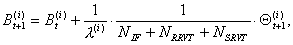(2)

where Θt+1(i) is the total time-t+ excess order — the sum of all orders emitted in the time interval (t, t+1) — of bond i and λ(i) is a constant liquidity factor that accounts for the depth of the market in bond i. One disadvantage of this linear formulation is that prices can become negative, which could be avoided by using a log-price formulation for the price formation rule. Outstanding orders in any given trading interval are always filled at the quoted prices and the market maker absorbs the excess or covers the shortfall, adjusting the prices according to the impact function (2).

#### First and Second Order Trading Strategies

2.4
In our model, a strategy is a rule by which an agent determines the next-period order vector θt+1 based on the available information. Zero-order strategies are rules that take only information from the system environment into account. In the context of financial markets, zero-order strategies may not seem particularly interesting but they are exemplified in decisions where factors such as macro-economic variables play a role. However, traders in our model will not make use of zero-order strategies. Second-order strategies, in contrast, are rules that depend not only on information from the environment, but also on agent-specific characteristics, such as the type of valuation models that competitors use. The most pervasive strategies are first order however, and rely on aggregates such as price or volatility to determine trade orders.

2.5
In the following sections we will discuss the three trader types and their strategies in more detail. Investment funds, which are basically fundamental traders, believe that prices follow a value process driven by exogenous factors. Reactive relative value traders, which are technical traders, believe that future prices can be extrapolated from past ones. Hence, these agents use first-order valuation strategies. Strategic relative value trader, in contrast, use second-order strategies based on privileged information about the valuation and risk models used by investment funds and reactive relative value traders.
##### Investment Funds (IFs)

2.6
Investment funds belong to the class of fundamental traders, who focus on the intrinsic or economic value of financial securities rather than their historical price patterns. In our model, each investment fund IFf updates the perceived fundamental value of the bond i according to an exogenous random process of the form(3)

where the ε(i,f) are drawn from the normal distribution N(i,f)(i,f)) with constant but agent-specific drift and variance. Investment funds derive their next-period orders from a value measure based on the moving average(4)

2.7
The initial value ν0(i,f) is set to the price B0(i) of bond i. Using a moving average to model the value dynamics later allows the SRVTs to make reasonably accurate value forecasts (see 2.12). Investment funds emit orders proportional to the difference of actual price Bt(i) to the average fundamental value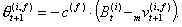(5)

2.8
Additionally, the trader's order is weighted by a capital factor c(f) that is either constant or inversely proportional to a measure of regret (see 2.19) so as to penalise underperformance. Once the investment funds have calculated their bond orders for the current trading interval, portfolio positions in both bonds are updated according to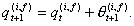(6)

In 2.21, we will introduce VaR risk limits for the portfolios, which allow us to restrict the total exposure of investment funds to price movements in bond i.

##### Reactive Relative Value Traders (RRVTs)

2.9
Reactive relative value traders are a special creed of momentum or technical traders who use a simple strategy based on the change in the bond price spread st = Bt(1)Bt(2). Similar to investment funds, the relative value trader (RRVT) places orders that are proportional to a capital factor c(r) that is either constant or reflects the trader's idiosyncratic risk aversion and the confidence placed into the relative value strategy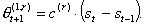(7)

2.10
Relative value trader engage in a zero cash strategy so that the order of the second bond is given by(8)

2.11
Once the RRVT has determined the orders for both bonds, total portfolio positions are updated according to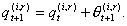(9)

##### Strategic Relative Value Trader (SRVT)

2.12
Strategic relative value traders base their strategy on a forecast of orders placed by investment funds and reactive relative value traders. In this model, the SRVT has knowledge of the past orders &thetak(i,f) placed by investment funds and the capital factor c(f) each fund is using in their strategy. Given the past orders, the SRVT can infer the values νk(i,f) for k < t+1 and hence calculate the expected value of the investment funds' MA(m) indicators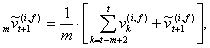(10)

where the 'tilde' denotes a random variable. Taking expectations on both sides of expression (10) then leads to the MA(m) estimate used by the SRVT to calculate the orders issued by the investment funds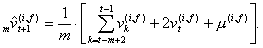(11)

2.13
The estimated total order issued by the investment funds is then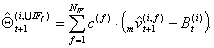(12)

2.14
Subsequently, the strategic relative value trader calculates the orders placed by the RRVTs in the current time period, which, knowing their capital factors can be determined exactly using (7) and (8). The total amount of bond i ordered by the RRVTs is(13)

2.15
In those simulation experiments where agents use VaR risk limits (see 2.21 for details), order constraints can apply which the SRVT has to take into account when estimating the orders placed by IFs and RRVTs. In that case, the SRVT, after estimating the next-period position of the IF or the RRVT, has to determine whether a risk limit is likely to apply or not, based on the next-period forecast of portfolio volatilities.

2.16
Once the current period forecasts of the orders for both the investment funds and the reactive relative value traders are determined, the SRVT will proceed to estimate the next-period prices using the price formation rule introduced in 2.3(14)

and, after applying an error correction, we obtain the final price estimate for both bonds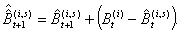(15)

2.17
The strategic relative value trader then issues orders for bond B(1) and B(2) based on the same spread momentum strategy used by the RRVTs, but with a next-period spread based on the bond price forecast (15)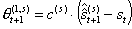(16)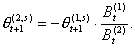(17)

2.18
The next-period portfolio position of the SRVT is thus(18)

#### Regret Adjustment of Agent Strategies

2.19
The market dynamics resulting from the trading activities of IFs, RRVTs, and SRVT is analysed in detail in 3.3 and 3.6 for the different strategies discussed above. Up to this point, agents invested into trading opportunities independently of their actual success or failure. Although in our model traders are not allowed to choose alternative strategies in case of losses, they are allowed to reduce their exposure if there is a significant risk of loss. To model this behaviour, we adjust the hitherto constant capital factor with an expected regret measure of the form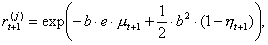(19)

where μt+1 is the mean of total accumulated profits, e is a scaling factor, ηt+1 is the confidence level for the chosen strategy — see equation (22) — and b is a measure for the investor's risk aversion. In the following, we take the constant capital factor to represent the total capital available to the investor. At each trading interval the expected regret measure r allows traders to determine the amount of capital they are prepared to invest into the trading opportunity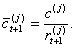(20)

2.20
In order to determine the regret measure (19), trader Aj first calculates the accumulated time-t profit of her portfolio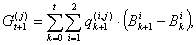(21)

and then determines the confidence level of the strategy by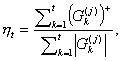(22)

where (G)+ = max(0, G).

#### Portfolio VaR Limits

2.21
What is the effect that risk management models such as VaR have on the profitability of the SRVT strategy? Are VaR position limits likely to induce irregularities into the price dynamics and reduce the chances of the SRVT to make a profit? Experiments E2.3 and E2.4 try to answer these questions. The following section describes the VaR model used in the investors' decision making process.

2.22
Up to this point, agents exclusively relied on valuation models to determine the number of bonds to buy or sell in any given trade interval and considerations of risk only entered via the variable capital factor. To account for the market risk of the bond portfolio, we now introduce a risk model based on VaR position limits. Value-at-Risk is a widely employed tool to manage market risk of an investment portfolio based on the positions, volatilities, and correlations of the portfolio assets. We assume that for a given portfolio and time horizon Δth = tht the P&L ΔVt is distributed normally with mean μ = 0 and normalised variance (σ t)2. An estimate for the maximum loss of the portfolio value for the confidence interval ηVaR is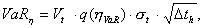(23)

such that ProbV(th) < VaRη} > ηVaR. After calculating the orders θt(i,j), the time-t change of the portfolio value for agent Aj is equal to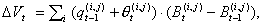(24)

with ΔVt = Vt — Vt-1. Given a maximum loss limit L(j) for agent Aj, the upper bound on the time-t value of the portfolio is then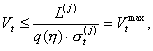(25)

where a confidence level of 99% or, equivalently, q(η) = 2.33, is used. In case condition (25) is not met, the trader will reduce the time-t orders to a level where the VaR risk limit condition is satisfied and the proportion of the bonds in the portfolio remains unchanged. Suppose now that Vt exceeds the maximum permitted portfolio value and that the difference between both values is given by δVt. Furthermore assume that time-t orders are reduced proportionally with the factor d = q(2)/q(1). It follows then that orders need to be reduced by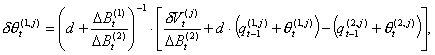(26)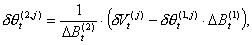(27)

so that the portfolio satisfies the VaR risk limit. To calculate the volatility of the two-bond portfolio σ t, the historical correlation between both bonds has to be taken into account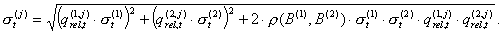(28)

2.23
Here qrel(i) is the relative size of the position of asset i, and σ t is the time-t bond volatility calculated over a window of size z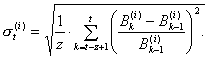(29)

The correlations are calculated using a window of the same size.

###Market Simulations

3.1
We can now turn to the question of how simulations of valuation and risk models might help sophisticated market players such as our strategic relative value trader to create trading strategies that can generate excess profits compared to strategies based on historical price patterns only (used by the RRVTs). In the following discussions, two sets of simulation cases illustrate the effectiveness of higher-order strategies under varying market conditions. In particular, we analyse the profitability of these strategies in situations where market participants use VaR risk limits and compare them to scenarios where no risk limits apply. In the first set of simulation experiments (Case 1), we evaluate the profitability of the fundamental and the reactive relative value strategies in the absence of the more sophisticated strategic relative value traders. In the second set (Case 2), we add a SRVT who uses a model that incorporates information about the models used by the other investors.

3.2
To avoid an excessively lengthy description of results, only a brief overview of Case 1 results is given here. A complete account together with a more detailed discussion can be found in Appendix A. In the remaining sections we will focus more extensively on Case 2, analysing the effects of incorporating a strategic relative value trader into the 'standard' investor population.

#### Case 1: A Population of Investment Funds and Reactive Relative Value Traders

3.3
In the first set of simulation experiments (Case 1), we consider a trading population made up of investment funds and reactive relative value traders. Each experiment, or simulation run, consists of 200 trading intervals where agents place bond orders in accordance with their respective valuation and risk models. The simulation-dependent parameters for Case 1 are listed in table 1. All other parameters are fixed and are listed in Appendix B. Moreover, we are using identical random number sequences across the different simulation experiments so that the results become comparable.

3.4
In our artificial market, 20 investment funds and NRRVT reactive relative value traders buy and sell two bonds that are in infinite supply. Market prices for these bonds are set by a market maker depending on the size of the total order excess or shortfall. Each investment fund receives an exogenous, private signal and updates the perceived fundamental value of the two bonds accordingly. The signal for each IF is drawn from a normal distribution where both the mean and standard deviation are different for each agent.
 Table 1: Parameter settings for Case 1 Experiment λ(1), λ(2) NRRVT VaR E1.1 1.1 1 no E1.2 4 1 no E1.3 1.1 20 no E1.4 1.1 20 L(j) = 0.5
VaR: agents using VaR position limits

##### Overview of results

3.5
We ran a series of four experiments for Case 1 to analyse how a variation in several crucial parameters affects the market dynamics and the performance of the fundamental and relative value strategies. The following brief discussion summarises our findings as to the effects of liquidity, number of relative value traders, capital factor, and VaR risk limits, ceteris paribus, on the performance of fundamental and relative value strategies. The details of Case 1 can be found in Appendix A.
• Variation of liquidity. When liquidity decreases, prices follow more closely the random walk of the exogenous value process. This situation where asset prices change erratically benefits the investment funds, since prices tend towards the (average) forecast value allowing the IF population to accumulate large net profits in aggregate. The spread momentum (RRVT) strategy in contrast fails to produce any significant gains and accumulated profits show a clearly downward trend. However, when liquidity increases, the price process becomes much smoother, which considerably improves the accuracy of the forecasts of the momentum strategy for next-period prices. Results show much improved levels of confidence and accumulated profits for relative value traders.
• Variation in NRRVT. When investment funds are in the majority compared to relative value traders, the fundamental strategy determines price behaviour. In that case, prices follow closely the random average value process of IFs. As we have seen in the case of low liquidity where prices closely follow the value process, trading on fundamentals is the superior strategy. In that case, the fundamental strategy of investment funds pays off handsomely (in aggregate), resulting in a pronounced upward trend of average profits, while momentum traders are loosing money. It is important to understand however that since accumulated profits have been averaged over all investment funds, it tells us only half the truth. Looking at individual IFs, the confidence levels in the fundamental strategy are quite close to 0.5, which means that aggregate IF profits are not a good measure for individual profit making in the long run.
• Constant vs. variable capital factor. A variable capital factor — rather than a constant one — allows agents to switch from a single-strategy to a continuous strategy regime and to choose a portfolio composition that best suits their risk profile. When both investment funds and relative value traders use a variable capital factor, relative value traders become much more confident in using the momentum strategy, with confidence levels consistently exceeding 0.5. In that case, prices do not follow the value process as closely as they did for constant capital factors, and bond price volatility is significantly reduced. As a result, more stable price patterns emerge that improve the profit outlook of relative value traders.
• Use of VaR risk limits. When investment funds use a value-at-risk (VaR) methodology to limit their portfolio position in the two bonds, price volatility increases well beyond that experienced in the previous experiments whenever risk thresholds are set to low values. This is so because as soon as prices pass the threshold, investment funds sell off part of their asset holdings to reduce their market exposure, thereby sending prices down a spiral that triggers further sales. Under this scenario, bond spreads can change abruptly to the disadvantage of the RRVTs, who fare very poorly in such an environment.

3.6
In the second set of simulation experiments, we add an additional trading agent who uses a higher-order relative value strategy to trade on privileged knowledge about the models reactive relative value traders and investment funds use to determine their orders. Each of the five experiments E2.1 — E2.5 involves 20 investment funds, NRRVT reactive relative value traders, and a single strategic relative value trader.
 Table 2: Parameter settings for Case 2 Experiment NRRVT m VaR SRVT knows about VaR? E2.1 1 1 no N/A E2.2 20 15 no N/A E2.3 20 15 L(j) = 0.5 no E2.4 20 1 L(j) = 0.5 yes E2.5 20 1 no, L(j) = 0.5 yes
m: moving-average window size; VaR: agents use VaR position limits

In each trading interval, bond prices are again set by the market maker according to the price impact function (2). Each experiment consists of 200 trading intervals, except for E2.5, where profits of the SRVT are compared with those of the RRVTs over a sample of 10,000 trading rounds, each consisting again of 200 trading intervals. The initial price for both bonds is (as in Case 1) B0(i) = 10, i = 1,2, the size of the volatility window for VaR calculations is z = 25, the drifts of the exogenous value processes are drawn from the uniform distribution U(-0.03,0.03), and the volatility is equal to 0.2. All experiments have been carried out with the liquidity factors λ(1) = λ (2) = 1.1. Simulation runs have been conducted with both a constant capital factor of 1 and with a regret-dependent factor as discussed in 2.19. The scaling factor e used in the expression for the expected regret has again been set to ln(0.001)/21/2 in all experiments.

##### One RRVT, small MA (m = 1), and no VaR (E2.1)

3.7
Parameters for this experiment are identical to those chosen in E1.1, with a difference however: a strategic relative value trader has been added to the market. The strategies of both RRVT and SRVT are fundamentally the same, but whereas the RRVT uses two past values of the bond price spread to calculate the next period order, the SRVT makes a prediction of price spreads based on superior information about the trading strategies of both the RRVTs and the IFs and uses this spread forecast to calculate the order size for both bonds. Results show that confidence levels of the SRVT are by a factor of 1.5 lower than those of the RRVTs in the case of a constant capital factor (c = 1). In this particular situation, with only one RRVT actively trading in the market and the capital invested in the strategy remaining time-invariant, confidence levels are very low, not exceeding 40% and therefore making both strategies unattractive.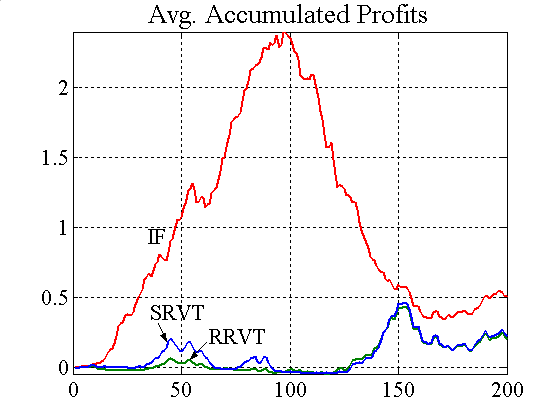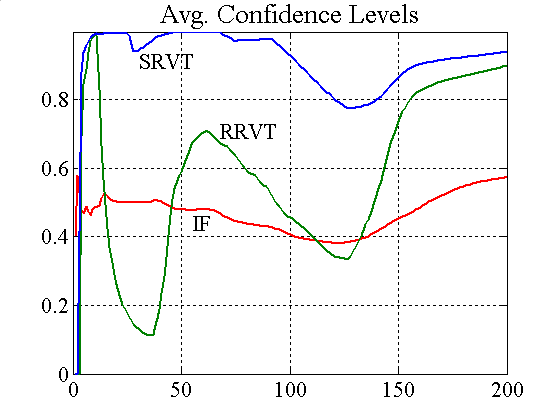Figure 1. Accumulated profits and confidence levels (variable capital factor)

In a market environment where investment funds outnumber RRVTs, the relative value trading strategy of either the RRVT or the SRVT shows very poor performance levels, not unlike those seen in Case 1. Since the prices follow closely the random walk of the fundamental values, there is not much room for manoeuvre for relative value traders to exploit the direction of spread movements. Figure 1 shows that the SRVT nevertheless has a slight advantage over the RRVT and that confidence levels in the higher-order strategy are well above 0.8. Hence, despite the average accumulated profits of the relative value strategy being lower than of the investment strategy, the small profit made by the SRVT is more certain. As noted above, accumulated profit graphs only show average values, and profits do change considerably from one investment fund to another. Since confidence levels of IFs are only slightly higher than 0.5, the fundamental strategy is equivalent to a zero-sum game.

##### Several RRVT, large MA (m = 15), and no VaR (E2.2)

3.8
We now analyse the performance of the strategic trader when the RRVTs equal the IFs in numbers. We assume that investment funds forecast the bonds' fundamental value using a moving average window of m = 15. The increase in the MA window size will induce regularities in the price dynamics that the strategic trader can exploit. In fact (see figure 2), the price predictions of the SRVT, which are based on the knowledge of the valuation models used by other traders, are much more accurate than those of the RRVTs, who use a simple momentum strategy based on historical prices from the last two trading periods.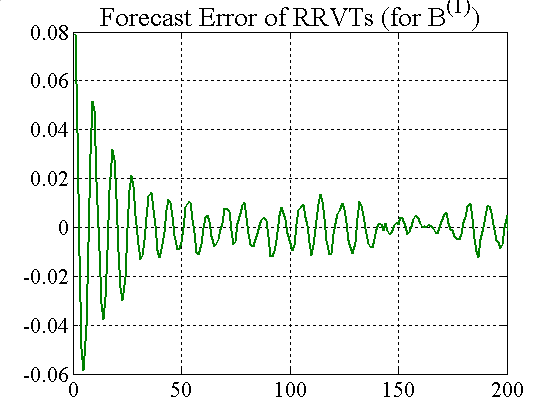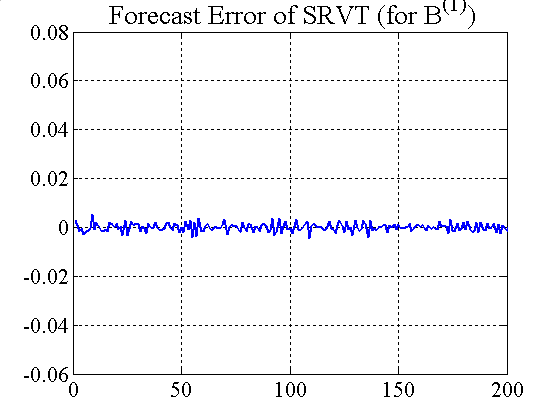Figure 2. Forecast error for RRVTs and SRVT (constant capital factor)

While the RRVTs do not make any substantial profit, the strategic trader does very well for both constant and variable capital factors, and the low prediction error results in steady gains, which in turn push confidence levels quickly to 1 (see figure 3). In this second case, where agents adjust their investment capital in accordance with the regret measure (19), the price behaviour becomes much less oscillatory, which is beneficial for the relative value traders, and in particular for the SRVT.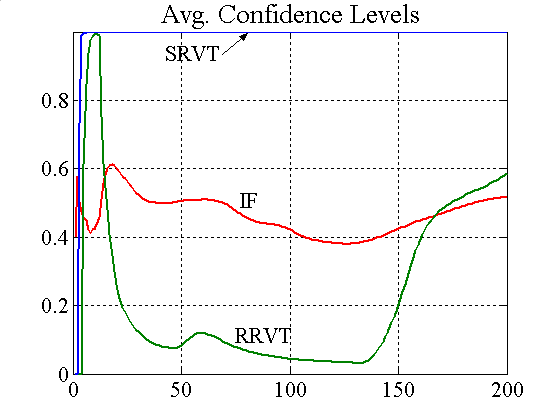Figure 3. Accumulated profits and confidence levels (variable capital factor)

##### Several RRVT, large MA (m = {1, 15}), and VaR Risk Limit (E2.3)

3.9
Experiment E3.2 studies the effect a VaR risk management model, used by the IFs, has on the price dynamics and the profitability of the fundamental and relative value strategies of both SRVT and RRVTs. As we have commented in Case 1 above, setting VaR limits to low values tends to result in volatile prices, which has a particularly nefarious effect on the profitability of the relative value trading strategy.Figure 4. Accumulated profits and confidence levels for variable capital factor (incl. VaR)

Figure 4 shows that the SRVT strategy results in persistent losses because of the abrupt price changes caused by the investment funds' VaR position limits, even though the IFs determine the bonds' fundamental value based on a moving average (note that when the IFs used a moving average with a window of m = 15 in experiment E2.2, this had a clear benefit for the profitability of the higher-order strategy of the SRVT).

##### Several RRVT, small MA (m = 1), and VaR Risk Limit (E2.4)

3.10
In experiment E2.4, the strategic relative value trader incorporates the knowledge of the investment funds' VaR model into her valuation model and the profitability of the trading strategy changes notably (see figure 5), and even when m = 1, the SRVT makes a profit. Figure 7 shows that the SRVT forecast errors are much smaller than those of the RRVT, and that the confidence levels for the higher-order strategy quickly tends to 1 (for both constant and regret-dependent capital factors — figure 6).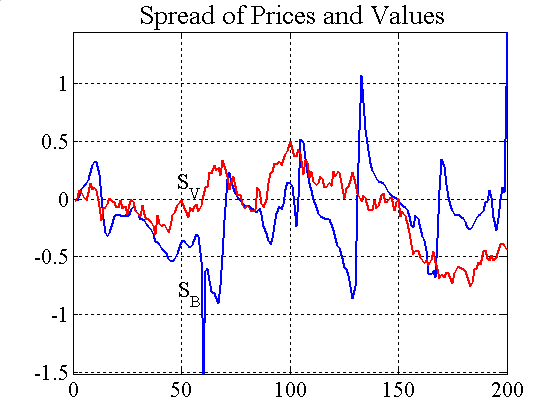Figure 5. Price spread and accumulated profits (variable capital factor)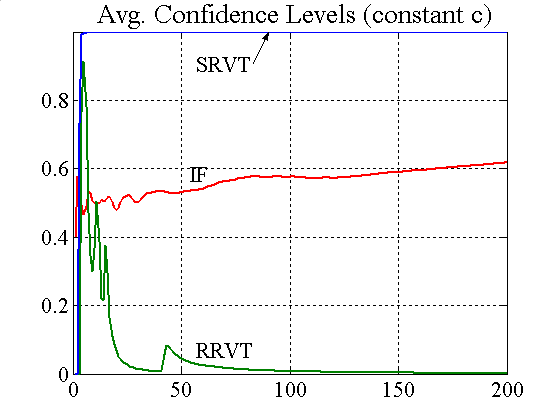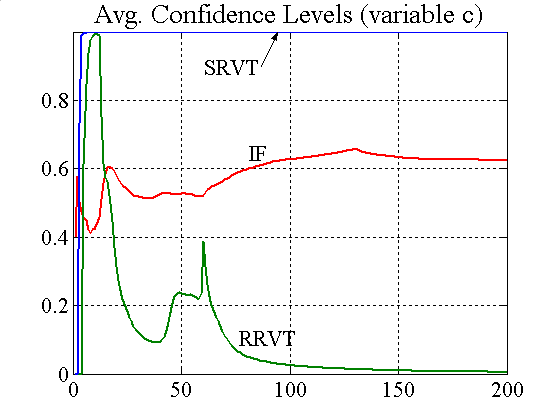Figure 6. Confidence levels for constant and variable capital factorFigure 7. Forecast error for RRVTs and SRVT (constant capital factor)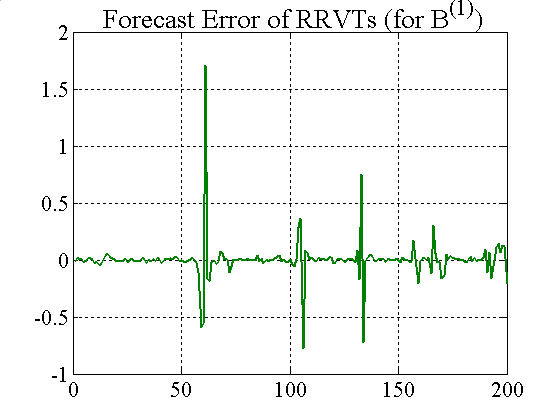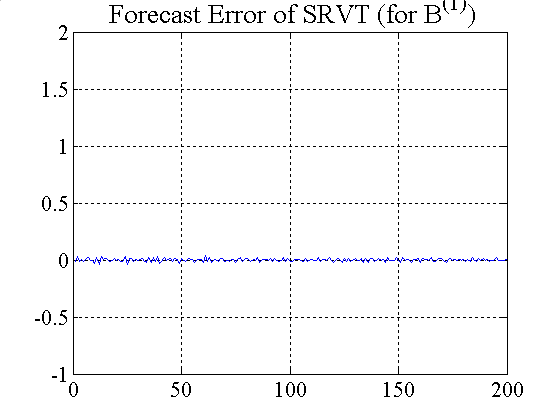Figure 8. Forecast error for RRVTs and SRVT (variable capital factor)

Although the forecast errors of the RRVT are generally small when using a variable capital factor (figure 8), they become very large when the investment funds adjust their holdings according to their VaR position limits (see figure 5).

##### Temporal and Cross-Sectional Analysis (E2.5)

3.11
We discuss now the results of a simple temporal and cross-sectional analysis that shows the relative performance of the reactive relative value strategy of the RRVT and the higher-order relative value strategy of the SRVT. In the first simulation run the investment funds do not use any VaR risk limits, whereas in the second run investment funds use a VaR risk model to limit their portfolio exposure.

3.12
A simulation run covers 10,000 trading rounds, consisting of 200 trading intervals each. There are 20 investment funds and 20 reactive relative value traders in the market. The liquidity factor is λ = 1.1 and the VaR limit is L(j) = 0.5 for the second simulation run where investment funds use a VaR position limit. The temporal histograms show the average differences of accumulated profits for SRVT and RRVT, calculated using equation (21) and averaged over the 20 RRVTs. This procedure is repeated for all profit path pairs of the 10,000 trading rounds. For the cross-sectional histograms, the differences of RRVT and SRVT profits are added at each time point over all 10,000 paths, and then averaged in all 200 time buckets.

3.13
Figure 9 clearly shows that the SRVT who uses a higher-order strategy consistently outperforms the RRVTs. The histogram of temporal differences brings the more relevant characteristic of profit differences to the fore. The difference between two individual profit paths can be thought of as a "strong" indicator of strategic superiority, since, unlike in the case of cross-sectional averaging, we compare two actual realisations of the profit process. The cross-sectional histogram in contrast gives merely a probabilistic picture — it is a "weak" indicator — of the strategic advantage of the SRVT, since it averages profit differences for all paths at each point in time. In the absence of VaR limits, price behaviour is less erratic and the advantage of the SRVT over the momentum strategy of the RRVT is only small. However, whenever investment funds use VaR models to set position limits, the SRVT strategy pays off considerably (see figure 9).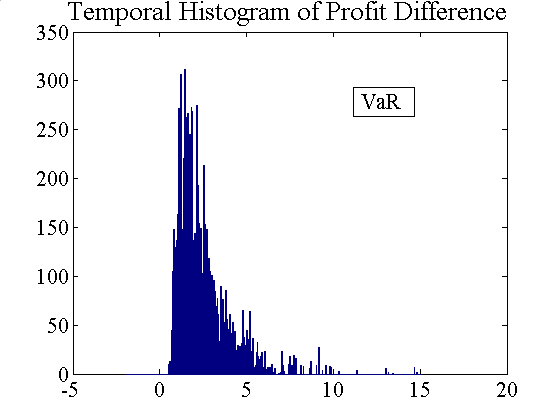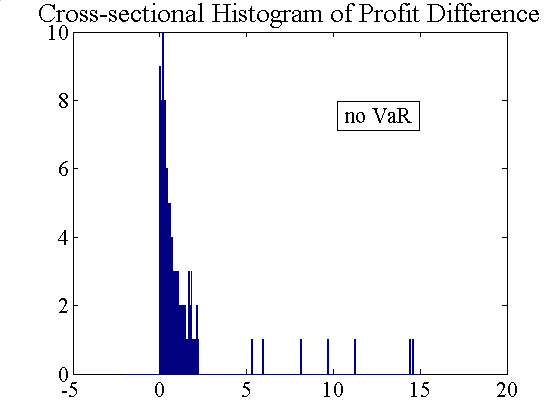Figure 9. Temporal and cross-sectional histograms of accumulated profit differences (left: without VaR, right: with VaR)

###Conclusions and Future Research

4.1
In this article we have shown how informed traders can devise superior strategies to exploit the performative nature of financial models. In particular, we introduced VaR models as a position limiting strategy for investment funds, and strategic relative value traders take advantage of price patterns induced by the use of VaR risk limits. We have demonstrated that in a stylised two-asset financial market in which the decision making process of fundamental and relative values traders is tightly linked to the use of valuation and risk management models, traders employing strategies that explicitly account for the use of these models can profit from the regularities in price movements.

4.2
We explored the implications of our market model using multi-agent based simulations. There are initially two types of traders in our market: investment funds and reactive relative value traders, which use a fundamental strategy and a technical strategy respectively to determine buying and selling orders. In conjunction with the simple linear price impact function (2), the combination of fundamental and momentum trading can be viewed as a minimal market configuration where a reasonable price dynamics emerges. The investment funds — or fundamental traders — employ private, stochastic information about the value of the two bonds to decide if these are overvalued or undervalued, and place their orders accordingly. Reactive relative value traders employ a simple spread momentum strategy to calculate their orders. Although the RRVT strategy on its own is self-fulfilling — it always forces prices to move into a profitable direction — this changes when investment funds with their fundamental strategy based on the stochastic value process enter the market. In an IF-dominated population, we find that the more volatile the price process, the worse the relative value traders fare. This has been demonstrated in Case 1, which is extensively discussed in Appendix A.

4.3
To test the hypothesis whereby model-induced price patterns can be exploited by higher-order strategies, we introduced a new trader type into our market population. Strategic relative value traders employ a strategy that is fundamentally the same than that of the reactive relative value trader, but instead of constructing a historical measure of spread momentum, the new strategy includes information about the other market players' valuation and risk management models, and hence constructs a momentum measure based on a forecast of market orders. In Case 2, we have seen in all of our experiments that the strategic relative value trader not only fares better in terms of absolute profitability, but also in terms of confidence she places in her strategy. In summary, the higher-order strategy benefits from an increase in the number of RRVTs, a better predictability of IF orders (stemming from an increase in the size of the moving average window), a variable capital factor, and a decrease in price stability through the use of VaR position limits when the use of VaR models is known to the SRVT.

4.4
Two directions of future research present themselves at this point. First, the strategy used by the SRVT has been chosen arbitrarily and switching between strategies is not possible, safe through an adjustment of the capital outlay via the regret measure. We need to understand better — always in the context of this stylised market with its particular price dynamics — how a successful strategy construction and selection process can be integrated in our model. In particular, the implications for the price dynamics of the base strategies employed by investment funds and RRVTs has to be better understood in order to gauge the results obtained after including a higher-order strategy. The higher-order strategies themselves have to be constructed in a systematic fashion and the SRVT needs to be equipped with a proper strategy selection mechanism. Second, the model used here is highly stylised, and in order to create a more realistic trading environment with heterogeneous and possibly (semi-)autonomous agents, we need to move away from the equation-based, analytically motivated evolutionary economics framework towards a more flexible, multi-agent systems framework which, although embracing working concepts from evolutionary economics, would also allow for a more effective representation of agent autonomy and sociality.

###Notes

1(MacKenzie 2006: 17)

2 The analogy with roulette wheels is not complete, however, since even in a perfectly competitive financial market the actions of market participants are constitutive of the outcome — the price in this case — unlike in the case of a roulette game.

3 Profit and loss

4 although the number of investment funds is equal to 20 in all experiments, we have verified that the results are robust in the range of 10-100 IFs

5 the order excess or shortfall is the sum of all orders in any given trade interval

6 Experiments E2.1 - E2.4 use the same underlying random number sequences to ensure that the results are comparable

7 the parameter values used for all figures are specified in Appendix B

8 We use the same number of investment funds throughout the cases, so as to compare the simulation results. However, we have verified that the results are robust for different numbers of IFs — from 10 to 100

9 the order excess or shortfall is the sum of all orders placed by traders at a given time

10 the parameter values used for all figures are specified in Appendix B

###References

ARTHUR W B, Holland J H, LeBaron B, Palmer R and Taylor P (1997) Asset pricing under endogenous expectations in an articial stock market. SFI Paper 96-12-093.

BIS (1999) A Review of Financial Market Events in Autumn 1998. Basel: Bank for International Settlements.

BROCK W A and Hommes C H (1998) Heterogeneous beliefs and routes to chaos in a simple asset pricing model. Journal of Economic Dynamics and Control, Vol. 22, pp. 1235-1274.

CHIARELLA C (1992) The dynamics of speculative behaviour. Annals of Operations Research, Vol. 37, pp. 101-123.

DIAMOND D W and Dybvig P H (1983) Bank runs, deposit insurance, and liquidity. Journal of Political Economy, Vol. 91 (June), pp. 401-419. Reprinted in Federal Reserve Bank of Minneapolis Quarterly Review, Vol. 24, no. 1, Winter 2000, pp. 3-13.

FARMER J D (1998) Market Force, Ecology and Evolution. Santa Fe Institute Working Paper no. 08-12-117.

HIRSHLEIFER D (2001) Investor Psychology and Asset Pricing. Journal of Finance, Vol. 56, pp.1533-1597.

HOMMES C H (2006) "Heterogeneous Agent Models in Economics and Finance." In: Tesfatsion L, and Judd K L (Eds.) Handbook of Computational Economics, Volume 2: Agent-Based Computational Economics, Amsterdam: Elsevier Science B.V.

IMF (1998) World Economic Outlook and International Capital Markets. Interim Assessment - December 1998, Washington D.C., International Monetary Fund.

KAHNEMAN D and Tversky A (2000) Choices, Values, and Frames. Cambridge: Cambridge University Press.

KIRMAN A and Teyssière G (2002) Microeconomic models for long memory in the volatility of nancial time series. Studies in Nonlinear Dynamics and Econometrics, Vol. 5, pp. 281-302.

LLACAY B and Peffer G (2004) Modelo evolutivo del impacto de técnicas VaR en los mercados financieros. Proceedings of the 7th Spanish-Italian Meeting on Financial Mathematics, Cuenca (Spain), July.

LEBARON B (2006) "Agent Based Computational Finance". In: Tesfatsion L, and Judd K L (Eds.) Handbook of Computational Economics, Volume 2: Agent-Based Computational Economics, Amsterdam: Elsevier Science B.V.

LUX T and Marchesi M (1999) Scaling and criticality in a stochastic multi-agent model of a nancial market. Nature, Vol. 397, pp. 498-500.

MACKENZIE D (2006) An Engine, not a Camera: How Financial Models shape Markets. Cambridge: MIT Press.

MACKENZIE D (2003) Long-Term Capital Management and the Sociology of Arbitrage. Economy and Society, Vol. 32, no. 3, pp. 349-380.

MADHAVAN A (2000) Market Microstructure: A Survey. Journal of Financial Markets, Vol. 3, pp. 205-258.

MANTEGNA R and Stanley H E (2000) An Introduction to Econophysics. Cambridge: Cambridge University Press.

MAYER M (1999) Risk Reduction in the New Financial Architecture. Public Policy Brief no. 56, The Jerome Levy Economics Institute of Bard College.

MORRIS S and Shin H S (2000) Market Risk with Interdependent Choice. Paper presented to the Conference on Liquidity Risk, Frankfurt (Germany), June.

MORRIS S and Shin H S (2003) "Global Games: Theory and Applications". In Dewatripont M, Hansen L and Turnovsky S (Eds.), Advances in Economics and Econometrics (Proceedings of the Eighth World Congress of the Econometric Society). Cambridge: Cambridge University Press.

SAWYER R K (2003) Artificial Societies: Multiagent Systems and the Micro-Macro Link in Sociological Theory. Sociological Methods and Research, Vol. 31, no. 3, pp. 325-363.

SHLEIFER A (2000) Inefficient Markets. Oxford: Clarendon Press.

TAKAHASHI and H Terano T (2003) Agent-Based Approach to Investors' Behavior and Asset Price Fluctuation in Financial Markets. Journal of Artificial Societies and Social Simulation, Vol. 6, no. 3. Available online on http://jasss.soc.surrey.ac.uk/6/3/3.html.

###Appendix A — Simulations Results for Case 1: A Population of Investment Funds and Reactive Relative Value Traders

A.1
In this first series of simulation experiments, we consider a trading population made up of investment funds and reactive relative value traders, in absence of higher-order strategies, which are discussed in E2.1-E2.5. Each experiment or simulation run consists of 200 trading intervals where agents place orders in accordance with their valuation and risk models. The simulation-dependent parameters for Case 1 are listed in table A1. Identical series of random numbers have been used for the different experiments so that the results become comparable.
 Table A1: Parameter settings for Case 1 Experiment λ(1), λ(2) NRRVT VaR E1.1 1.1 1 no E1.2 4 1 no E1.3 1.1 20 no E1.4 1.1 20 L(j) = 0.5
VaR: agents use VaR position limits

A.2
In our artificial market, 20 investment funds and NRRVT reactive relative value traders buy and sell two bonds that are in infinite supply, and market prices for these bonds are set by a market maker depending on the size of the total order excess or shortfall. Each investment fund receives an exogenous, private signal according to which it updates the perceived fundamental (or 'intrinsic') value of the two bonds. The signal is drawn from a normal distribution where both the mean and standard deviation are different for each investment fund.

A.3
The initial price of the two bonds is equal to 10, the size of the volatility window for the VaR calculations in experiment E1.4 is z = 25, the drifts of the agent-specific, exogenous value processes are drawn from the uniform distribution U(-0.03,0.03), and the volatility is equal to 0.2 for all the processes. All experiments have been carried out with a constant (c = 1) as well as with a regret-dependent capital factor (see 2.19 for a discussion of the capital factors). The scaling factor e for the expected regret calculation has been set to ln(0.001)/21/2 and the agent's risk aversion b is equal to 21/2 for all experiments. In Case 1, no moving average is used in the investment funds' fundamental value models (to this effect, the MA window size has been set to m = 1). It is worth noting that these parameters have been chosen so that the price dynamics shows a reasonable behaviour. Consequently no parameter settings have been included under which prices diverge, since our chief interest lies in understanding the impact higher-order strategies have in a non-pathological market environment.

#### One RRVT, Low Liquidity, and no VaR (E1.1)

A.4
The first experiment E1.1 shows the difficulties that relative value traders have in making profits using a momentum-based spread strategy and when prices change erratically. We can create such a highly volatile market environment by setting the liquidity factor for both bonds to a relatively low value (see figure A1). Since investment funds are in an overwhelming majority compared to the single relative value trader, prices closely follow the random walk of the exogenous value process and bond price spreads consequently show a similar behaviour.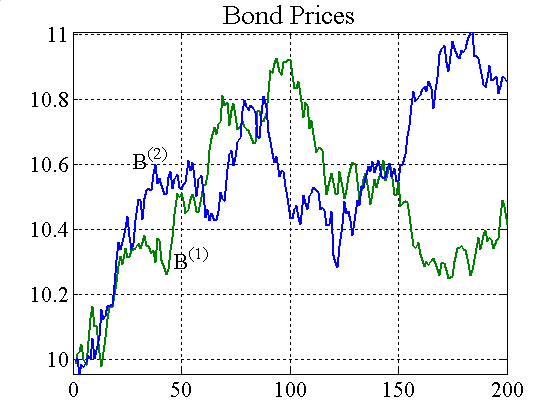Figure A1. Bond prices and price spreads for IFs and RRVTs

A.5
When prices change erratically, the spread momentum strategy usually fails to produce significant gains (unless prices exhibit trend behaviour) and accumulated profits show a clear downward trend (figure A2). The same profit graph seems to suggest however that the fundamental strategy employed by investment funds pays off handsomely, with a pronounced upward trend in average profits. However, the crux of the matter is that accumulated profits are averaged over the 20 investment funds, and this aggregate only tells half of the story in the case at hand. In fact, if we look at the volatility of accumulated profits (figure A3), we can clearly recognise the extreme variability of profits stemming from the fundamental strategy. To obtain a different perspective on the quality of investment fund profits, figure A2 shows the confidence levels for the strategies employed by both the RRVT and the IFs. As one would expect, the relative value trader quickly looses confidence in his ability to make a profit based on a momentum strategy, and confidence levels tend to zero after only a few trades. Although investment funds do not loose confidence in their fundamental strategy the same way, they settle at a confidence level of 0.5, which means that they value the quality of their strategy as not being better than a zero-sum game. Hence, the upward trend in average accumulated profits for investment funds is a misleading indicator for the performance of the fundamental strategy, since for individual traders large gains are likely to be followed by large losses and vice-versa, which makes the highly risky nature of this strategy visible.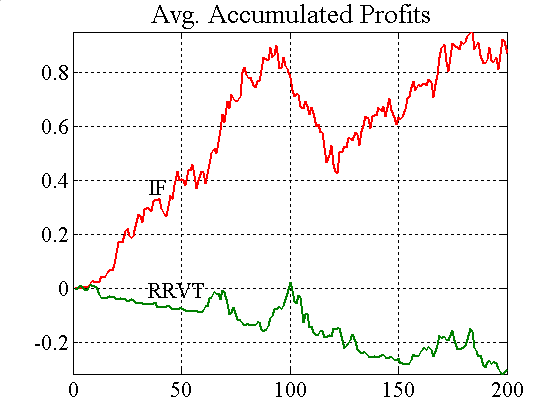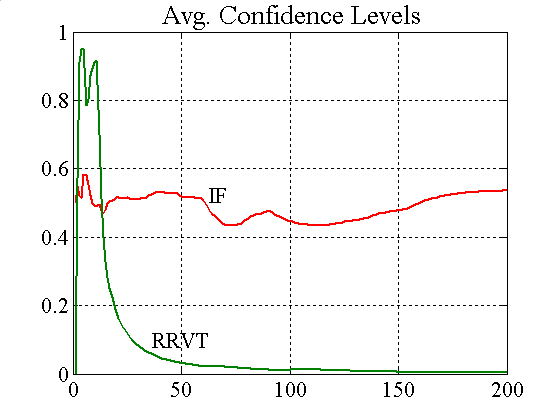Figure A2. Accumulated profits and confidence levels for IFs and RRVTs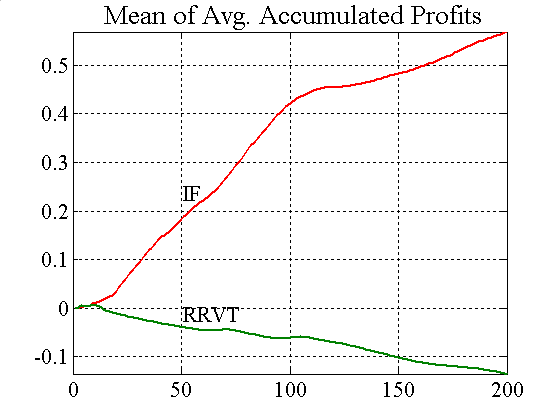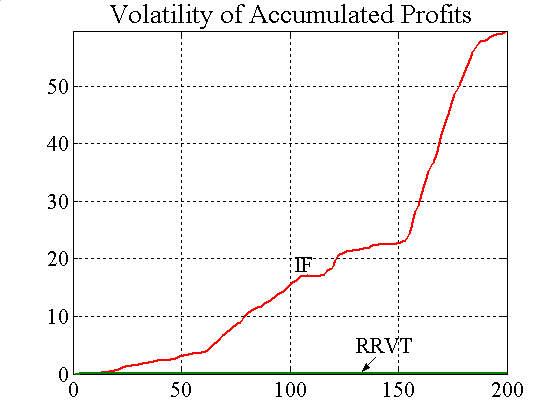Figure A3. Mean and standard deviation of accumulated profits for IFs and RRVTs

A.6
Up to this point, traders have been using a constant capital factor so that the amount they are investing is independent of the strategy's performance. In 2.19, we discussed a performance-dependent measure that agents can use to continuously adjust the invested capital depending on the profits generated by their trading strategy. By using a variable capital factor, agents are in fact switching from a single-strategy regime to a continuous strategy space where they select those strategies that best suit them at any given time. If for instance a chosen strategy fails to perform well in the market, agents adjust their capital investment so as to avoid larger losses, which can be interpreted as selecting a new strategy from a continuous scale. The impact that the use of a variable capital factor has on the portfolio performance of investment funds and relative value traders concludes our discussion of the results for experiment E1.1.

A.7
When both investment funds and relative value traders base their trading decisions on a variable capital factor, outcomes differ significantly — in certain aspects at least — from those obtained with a constant capital factor. In summary, relative value traders become more confident of their momentum strategy, reaching levels of confidence higher than 0.5. Looking at the price time series for both bonds in figure A4, we observe that prices do not follow the value process as closely as they do for a constant capital factor. Variability is significantly reduced in some time intervals (note for instance the marked decline in both prices between simulation step 130 and 160), which helps relative value traders to work their way out of the losses that they are accumulating in the more volatile regions. We can also observe a drop in volatility of investment fund orders when using a variable capital factor (see figure A5), and the emergence of regions showing persistence of buying or selling orders.Figure A4. Price and value process for both bonds

A.8
The results indicate that the use of a variable capital factor is an important instrument in creating a more stable price behaviour. In addition, it avoids the situation where relative value traders keep investing in a loosing strategy. Now, invested capital is reduced as soon as losses accumulate and persistent directional changes in spreads — beneficial for the relative value strategy — can now generate sufficient incremental gains for the strategy to become profitable. Figure A6 is a clear indication that the variable capital factor produces a qualitative shift in confidence for the relative value strategy, although still not sufficiently so. Confidence levels for this strategy are levelling off at 0.5 and are therefore comparable in its performance to a zero-sum game.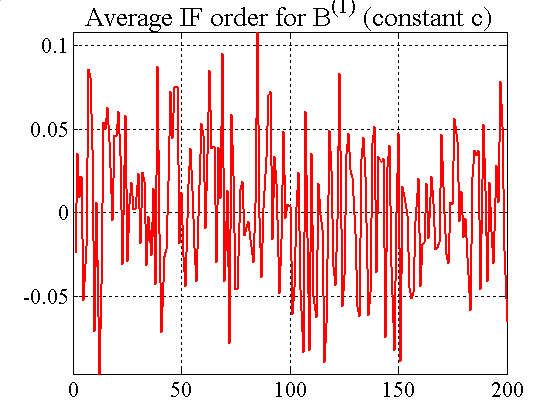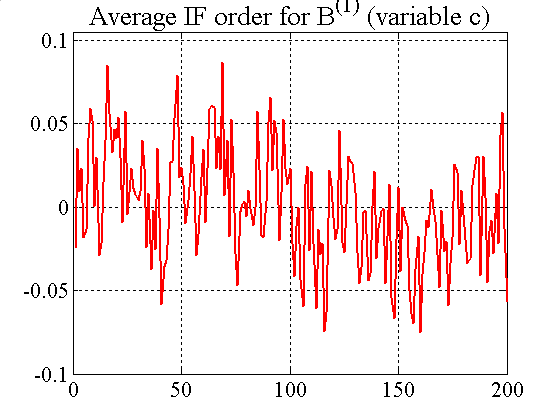Figure A5. Shortfall and excess order clustering for IFs

A.9
A comment regarding certain aspects of the price dynamics shown in figure A4 is pertinent at this point. How is it that, quite unlike in the case of a constant capital factor, regions of directional persistence in price movements now emerge, when the underlying value process actually shows higher variability? In a rising market, investment funds that have consistently believed that a bond is undervalued will have entered long positions, which in turn will generate substantial profits for them. This in turn leads to a heightened confidence in their strategy and therefore results in even larger positions. Investment funds believing, in the same rising scenario, that the asset is overvalued, will have entered a short position in the hope to make a profit when the prices eventually return to their fundamental levels. Since in the meantime prices continue to rise, these funds will loose money and therefore reduce the capital they invest into the fundamental strategy. As shown for bond B(1) in figure A4, the influence of the longs in such an environment can be so strong that prices are pushed far beyond average valuation levels. Upward movements in prices increase demand in the bond by longs more than downward movements can increase the supply by shorts because of the asymmetry created by the different capital factors applied by longs and shorts. Short-lived downward moves therefore do not alter significantly the confidence of longs in their strategy despite of temporarily making a loss. If the downward pressure persists however, longs with substantial positions will end up reducing exposure of their portfolio, and their influence on prices will fade as a consequence. Confidence of shorts in contrast will recover and the impact of their trades on prices will grow.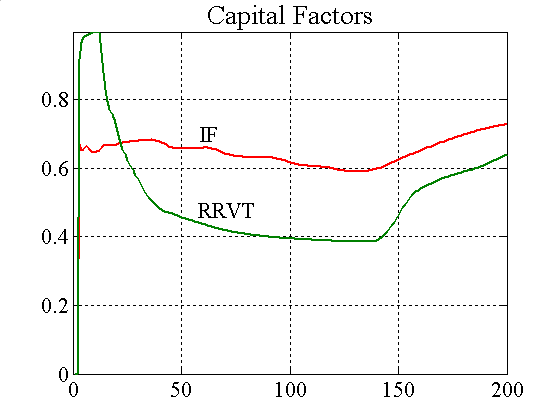Figure A6. Average confidence levels and capital factors for IFs and RRVTs

A.10
Comparing the RRVT confidence levels for a constant capital factor (figure A2) with those obtained for regret-adjustment (figure A6), the proportion of positive payoffs noticeably increases for the relative value strategy to the point where it reaches and surpasses the average level of investment funds. As we have already mentioned before, this is in stark contrast to the rapidly declining levels of confidence when RRVTs use a constant capital factor. Although the RRVT still makes losses at the beginning of the trading round, these do not persist as happens in the case of constant capital factor, and profits start recovering after 140 trading intervals. The dynamics of the capital factors for the individual investment funds is qualitatively similar to that of the RRVT, but as figure A7 shows, the pronounced shifts in the investment fund's capital factors are averaged out into a relatively stable aggregate.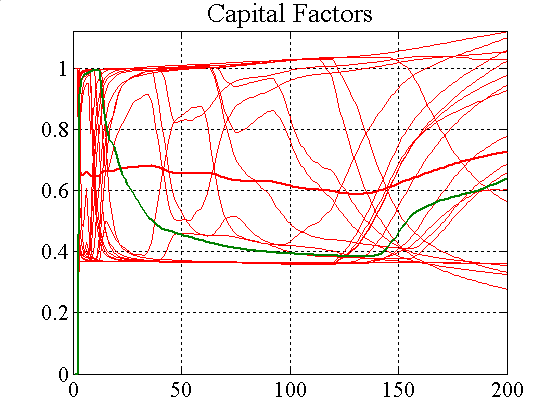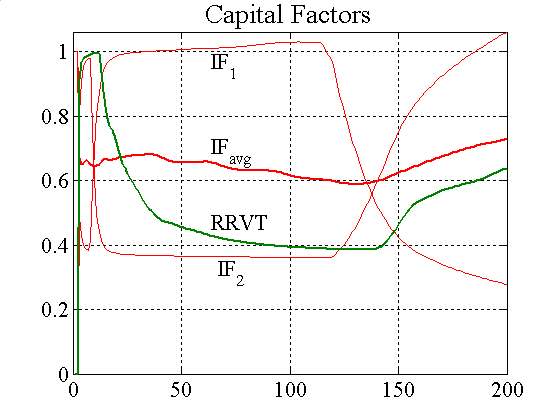Figure A7. IFs and RRVT capital factors and two individual paths

#### One RRVT, High Liquidity, and no VaR (E1.2)

A.11
In the previous experiment E1.1 the liquidity factor was set to low values to emulate a volatile market environment. As a result, relative value traders were unable to use their momentum strategy to produce any significant gains, and their strategy quickly led to large losses when using a constant capital factor. We now analyse the performance of the relative value strategy in a calmer market, where an increase in the liquidity factor of both bonds from λ = 1.1 to λ = 4 keeps volatility at low levels. The direct effect of higher liquidity is a smoother price dynamics, which considerably improves the accuracy of the momentum strategy forecast for next-period spreads (see figure A8 below). Even a cursory comparison of maximum absolute errors shows that these are approximately five times higher in the low-liquidity scenario than in case of λ = 4. Note that in the previous experiment, the use of a constant capital factor was detrimental to the performance of the RRVT, whereas here the relative value strategy shows much improved levels of confidence with no marked downward trend in accumulated profits (see figure A9).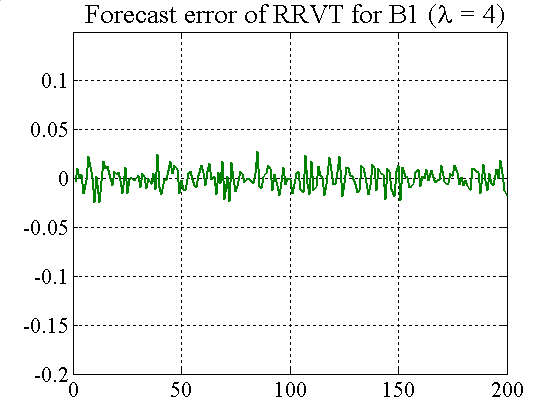Figure A8. Comparing forecast errors of RRVT for the two cases of λ = 1.1 and λ= 4 (constant c)Figure A9. Accumulated profits and confidence level for λ = 4 and constant capital factor

#### Several RRVTs, Low Liquidity, and no VaR (E1.3)

A.12
The previous experiment has shown how an increase in liquidity can be beneficial for the relative value trader, a result that is to be expected given that the spread momentum strategy works better in a calm market environment when price movements are less erratic. In the third experiment we analyse the performance of the relative value traders when they equal the IFs in numbers, and when the capital they invest in the momentum strategy is comparable to that used up by the fundamental strategy. Hence, we increase the number of reactive relative value traders to 20 and reset the liquidity to its original value (λ = 1.1).

A.13
When agents use a constant capital factor (c = 1) performance is highly erratic, with prices over- and undershooting the value process (not shown here). The oscillatory behaviour tends to erode very quickly what little gains the RRVTs make, given that the naïve spread momentum strategy performs only well in relatively non-volatile markets. Similar to the findings of experiment E1.1, where liquidity was set to an equally low level, applying a variable capital factor improves the profit outlook for the RRVTs, with the difference here that average accumulated gains are consistently above zero and the confidence level for the RRVTs reaches levels closer to 1 than to 0.5 (figure A10).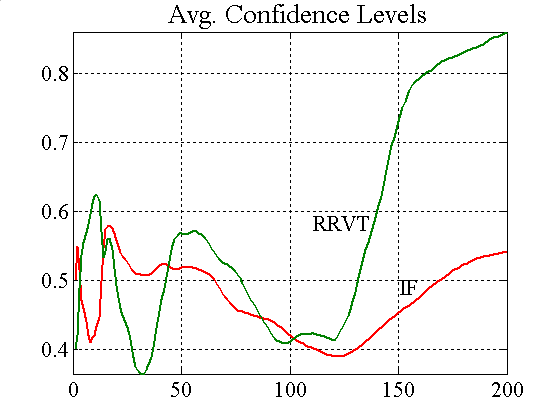Figure A10. Accumulated profits and confidence levels for variable capital factor and NRRVT =20

#### Several RRVTs, Low Liquidity, and VaR Risk Limit (E1.4)

A.14
The last experiment of Case 1 starts out from a volatile market environment with bond liquidities λ = 1.1. However investment funds use a value-at-risk (VaR) model to limit the portfolio position in the two bonds in accordance with a previously defined risk threshold. When the threshold is hit, orders emitted in any given trade interval are restricted so that the combined effect of price volatility and portfolio size does not cause unacceptable losses for the investor. By introducing a relatively low threshold for market risk, the price volatility increases well beyond the levels experienced in the previous experiment as VaR limits in our stylised market lead to heavy selling once they are exceeded (see figure A11). Here, bond spreads change abruptly and create a disadvantageous trading environment for the RRVTs, and even in the case of using a variable capital factor, the RRVTs are loosing out quickly (see figure A11).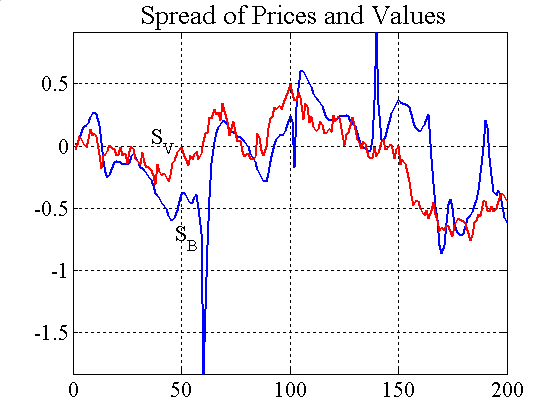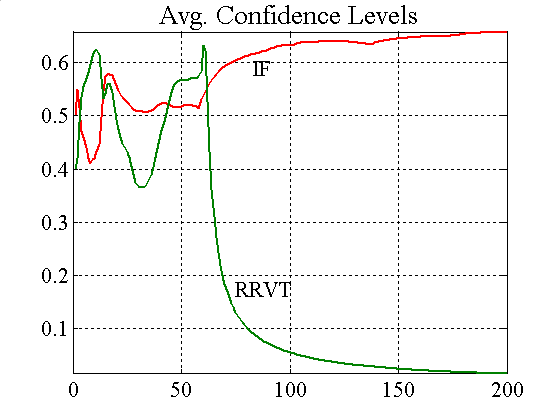Figure A11. Price spread and confidence levels when IFs use VaR

###Appendix B

 Table A2: Parameter Values Employed in the Figures Parameters Seed n B0(1), B0(2) λ(1), λ (2) z m NIF NRRVT NSRVT μ(1,f), μ(2,f) σ (1,f), σ (2,f) c? VaR? Fig. 1 21151 200 10 1.1 25 1 20 1 1 ~U(-0.03, 0.03) 0.2 variable no Fig. 2 21151 200 10 1.1 25 15 20 20 1 ~U(-0.03, 0.03) 0.2 const. no Fig. 3 21151 200 10 1.1 25 15 20 20 1 ~U(-0.03, 0.03) 0.2 variable no Fig. 4 21151 200 10 1.1 25 15 20 20 1 ~U(-0.03, 0.03) 0.2 variable L(f) = 0.5 Fig. 5 21151 200 10 1.1 25 1 20 20 1 ~U(-0.03, 0.03) 0.2 variable L(f) = 0.5 Fig. 6 21151 200 10 1.1 25 1 20 20 1 ~U(-0.03, 0.03) 0.2 const. / var. L(f) = 0.5 Fig. 7 21151 200 10 1.1 25 1 20 20 1 ~U(-0.03, 0.03) 0.2 const. L(f) = 0.5 Fig. 8 21151 200 10 1.1 25 1 20 20 1 ~U(-0.03, 0.03) 0.2 variable L(f) = 0.5 Fig. 9 N/A 200 10 1.1 25 1 20 20 1 ~U(-0.03, 0.03) 0.2 variable no / L(f) = 0.5 Fig. A1 21151 200 10 1.1 25 1 20 1 0 ~U(-0.03, 0.03) 0.2 const. no Fig. A2 21151 200 10 1.1 25 1 20 1 0 ~U(-0.03, 0.03) 0.2 const. no Fig. A3 21151 200 10 1.1 25 1 20 1 0 ~U(-0.03, 0.03) 0.2 const. no Fig. A4 21151 200 10 1.1 25 1 20 1 0 ~U(-0.03, 0.03) 0.2 variable no Fig. A5 21151 200 10 1.1 25 1 20 1 0 ~U(-0.03, 0.03) 0.2 const. / var. no Fig. A6 21151 200 10 1.1 25 1 20 1 0 ~U(-0.03, 0.03) 0.2 variable no Fig. A7 21151 200 10 1.1 25 1 20 1 0 ~U(-0.03, 0.03) 0.2 variable no Fig. A8 21151 200 10 1.1, 4 25 1 20 1 0 ~U(-0.03, 0.03) 0.2 const. no Fig. A9 21151 200 10 4 25 1 20 1 0 ~U(-0.03, 0.03) 0.2 const. no Fig. A10 21151 200 10 1.1 25 1 20 20 0 ~U(-0.03, 0.03) 0.2 variable no Fig. A11 21151 200 10 1.1 25 1 20 20 0 ~U(-0.03, 0.03) 0.2 variable L(f) = 0.5